# Box Muller Transform: Simple Definition

Share on

A Box Muller transform takes a continuous, two dimensional uniform distribution and transforms it to a normal distribution.

It is widely used in statistical sampling, and is an easy to run, elegant way to come up with a standard normal model. In fact, since it can be used to generate normally distributed random numbers, it was originally developed as a better and computationally efficient alternative to inverse sampling.

## Running the Box Muller Transformation

At its most basic, the Box-Muller transformations simply takes two variables that are uniformly distributed and sends them to two independent random variables with a standard normal distribution.

Let’s say U1 and U2 are our original independent random variables; they are uniformly distributed in the interval (0,1). The Box-Muller transformation creates new Z0 and Z1; independent, random variables that have a standard normal distribution: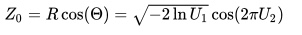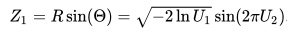The derivation here is based on the way we can represent any point in the X,Y Cartesian plane through polar coordinates, with a radius and an angle.

## The Polar Form of the Box Muller Transform

There’s another form of the Box-Muller transform which uses the Pythagorean identity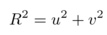as well as the identities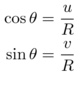to rewrite the above equations as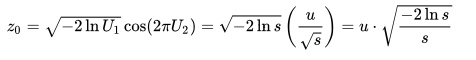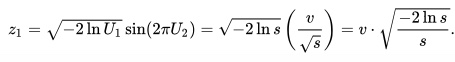Although the same points are being mapped to as with the ‘basic form’ of the Box-Muller transform, given above, this alternate form is sometimes preferred because it replaces sine and cosine with simple divisions and so is often more computationally efficient.

## References

Goodman, Jonathan. Lecture Notes on Monte Carlo Methods. Chapter 2: Simple Sampling of Gaussians Retrieved from https://www.math.nyu.edu/faculty/goodman/teaching/MonteCarlo2005/notes/GaussianSampling.pdf on March 16, 2018

Vafa, Keyon. The Box Muller Transform.